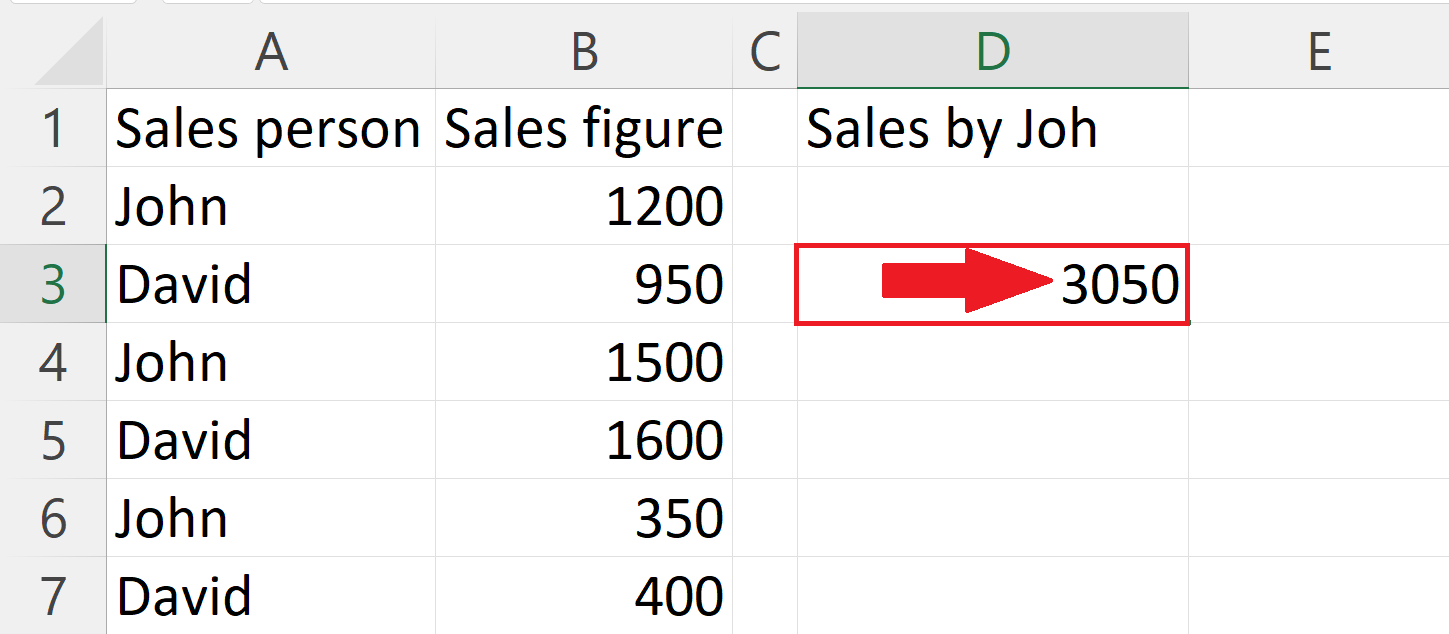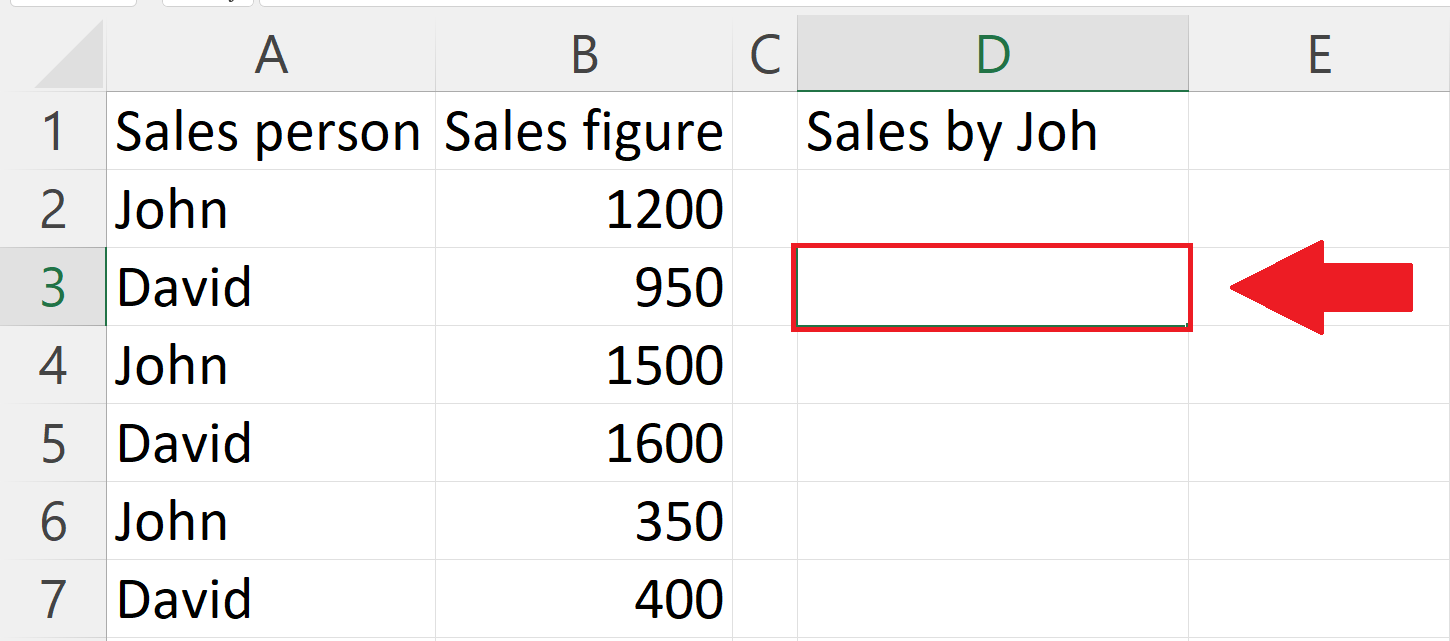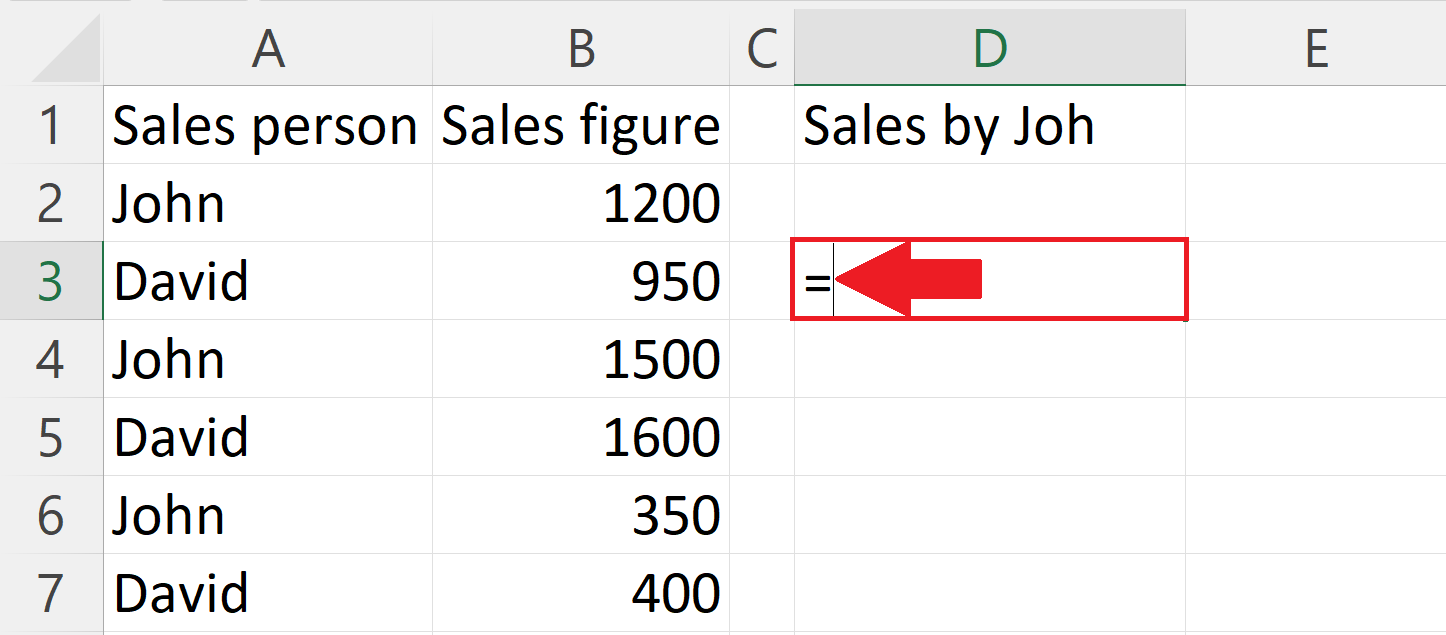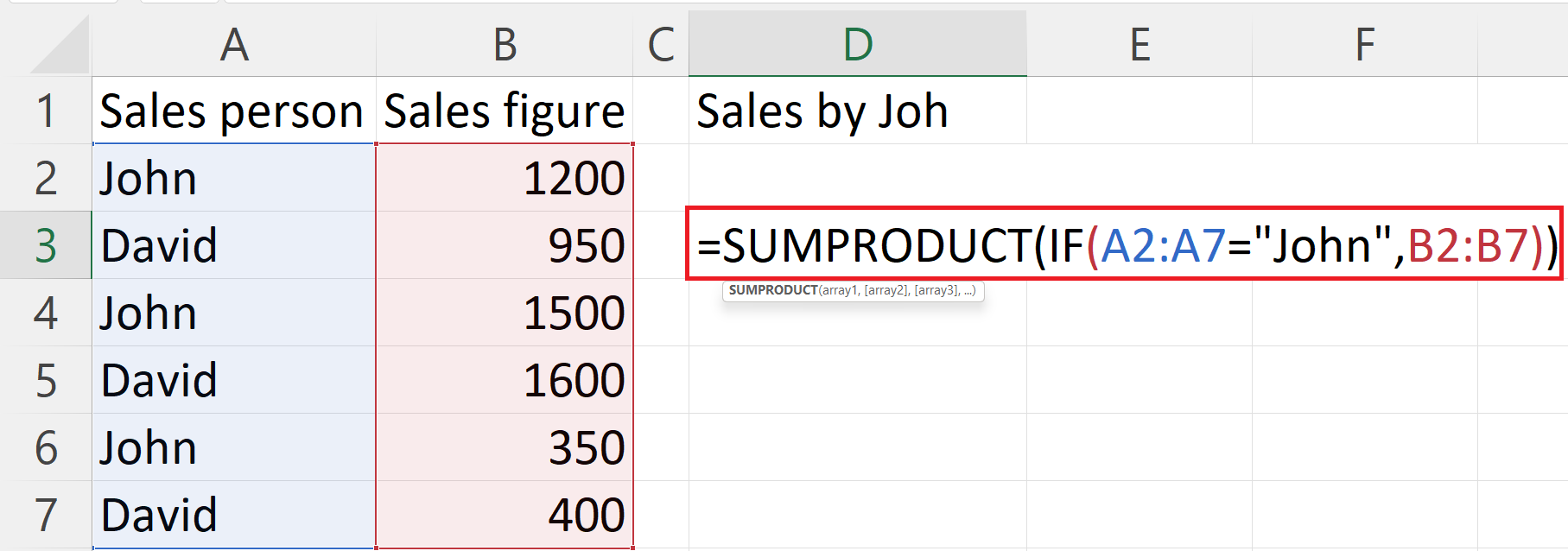# How to use SUMPRODUCT IF formula in Microsoft Excel

In  this tutorial we will learn how to use SUMPRODUCT IF formula in Microsoft Excel. The SUMPRODUCT IF formula in Microsoft Excel is a versatile and useful tool that combines the functionality of two different functions, SUMPRODUCT and IF. The SUMPRODUCT IF formula in Microsoft Excel is a combination of the SUMPRODUCT and IF functions, and is used to sum the product of values in a range of cells based on one or more conditions. The syntax for the SUMPRODUCT IF function is :  SUMPRODUCT(IF(criteria, [sum_range]))Microsoft Excel is a powerful spreadsheet software that is widely used in both personal and professional settings for a variety of purposes, including data analysis, financial modeling, and project management. With its versatile tools and functions, Excel allows users to organize, calculate, and visualize data in a flexible and efficient manner. One of the key strengths of Excel is its ability to perform complex calculations with ease, including basic arithmetic operations, financial functions, and statistical analysis. The software also supports a wide range of chart types, making it simple to create visual representations of data.

### Step 1 – Select a blank cell– Select a blank cell in which you want to use the SUMPRODUCT IF formula.

### Step 2 – Place an Equals sign### Step 3 – Use the SUMPRODUCT IF formula– Here we have a list of sales persons in column A, and the corresponding sales figure in column B. You could use the SUMPRODUCT IF function to calculate the total sales for a particular salesperson. The formula would be
=SUMPRODUCT(IF(A2:A7=”John”,B2:B7))

– A2:A7 is the  range of cells to be evaluated based on the “criteria”.

– A2:A7=“John” is the criteria i.e. condition that must be met in order to multiply and sum the values in the “sum_range”.

– B2:B7 is the sum_range i.e. the range of cells to be multiplied and summed, if different from the “range”.

### Step 4 – Press the Enter Key– Press the Enter Key to get the result.